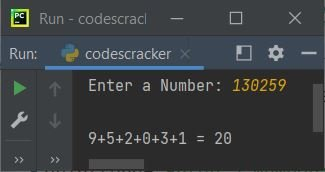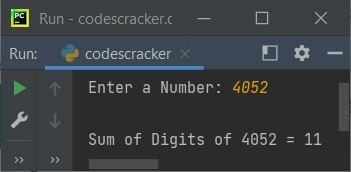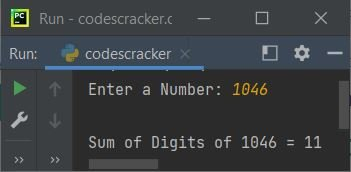# Python Program to Add Digits of a Number

In this article, you will learn and get code in Python, to find and print the sum of digits of a number entered by user at run-time. Here are the list of approaches used to do the task:

• Add Digits of a Number using while Loop
• Using for Loop
• Using Function
• Using class

For example, if user enters a number say 235, then the output will be 2+3+5, that is 10.

## Add Digits of a Number using while Loop

The program given below receives a number as input from user, and uses a while loop to find the sum of digits of given number:

```print("Enter a Number")
num = int(input())
sum = 0
while num>0:
rem = num%10
sum = sum+rem
num = int(num/10)
print("\nSum of Digits of Given Number: ", sum)
```

Here is the initial output produced by this Python program:Now supply the input say 123 and press `ENTER` key to find and print the summation of its digit 1, 2, and 3 as shown in the snapshot given below:The dry run of above program with user input 123 goes like:

• Since user enters 123, then it gets stored in num. Therefore num=123 (entered by user) and sum=0 (initialized 0 as its initial value)
• Now the condition of while loop gets evaluated. That is, the condition num>0 or 123>0 evaluates to be true, therefore program flow goes to its body and evaluates all the three statements
• So num%10 or 123%10 or 3 gets initialized to rem. Now rem=3
• And sum+rem or 0+3 or 3 gets initialized to sum. Now sum=3
• And finally int(num/10) or int(123/10) or int(12.3) or 12 gets initialized as new value of num. Now num=12
• Now the condition of while loop again gets evaluated. Because this time also the condition num>0 or 12>0 evaluates to be true, therefore again, all three statements gets executed. This process continues until the condition evaluates to be false
• In this way, after exiting from the loop, we'll have a variable named sum that holds 6, the sum of digits of given number
• Therefore just print the value of sum as output

#### Modified Version of Previous Program

This is the modified version of previous program. In this program, if user enters a number say 124, then output looks like 1+2+4=7

```print(end="Enter a Number: ")
num = int(input())

sum = 0
print(end="\n")
while num>0:
rem = num%10
sum = sum+rem
num = int(num/10)
if num==0:
print(end=str(rem))
else:
print(end=str(rem)+ "+")

print(" = " +str(sum))```

Here is its sample run with user input, 130259:Note - The end is used to skip adding an automatic newline using print().

## Add Digits of Number using for Loop

Now this program uses for loop instead of while to do the same task, that is to find and print the sum of given number's digits.

```print("Enter a Number: ", end="")
num = int(input())

sum = 0
temp = num

for i in range(len(str(temp)), 0, -1):
rem = num%10
sum = sum+rem
num = int(num/10)

print("\nSum of Digits of " +str(temp)+ " = " +str(sum))```

Here is its sample run with user input, 4052:Note - The third parameter of for loop (-1) is used to loop with loop variable (i) in reverse order. That is, the size of given number to 1 (one greater than 0 (the second parameter))

Since the length of "4052" (a string) is 4, therefore all three statements gets executed three times.

Note - The str() is used to convert an int value to a string type value

Note - The len() is used to find the length of string.

## Add Digits of Number using Function

This program uses a user-defined function named addNumDig() to find the sum of digits of a given number. The function receives a number as its argument and returns the sum of its digit.

```def addNumDig(n):
sum = 0
while n>0:
rem = n%10
sum = sum+rem
n = int(n/10)
return sum

print("Enter a Number: ", end="")
num = int(input())

print("\nSum of Digits of " +str(num)+ " = " +str(res))```

This program produces the same output as of previous program.

## Add Digits of Number using Class

This is the last program of this article. The program is created using class and object, an object-oriented feature of Python.

```class CodesCracker:
sum = 0
while n>0:
rem = n%10
sum = sum+rem
n = int(n/10)
return sum

print("Enter a Number: ", end="")
num = int(input())

ccObj = CodesCracker()

print("\nSum of Digits of " +str(num)+ " = " +str(res))```

Here is its sample run with user input, 1046:Using the following statement:

`ccObj = CodesCracker()`

All properties of the class named CodesCracker gets assigned to an object named ccObj. Now this object can be used to access the member function of class CodesCracker using dot (.) operator.

#### Same Program in Other Languages

Python Online Test

« Previous Program Next Program »